Courses

# Regular Expressions And Finite Automata MCQ Quiz - 2

## 20 Questions MCQ Test Mock Test Series - Computer Science Engg. (CSE) GATE 2020 | Regular Expressions And Finite Automata MCQ Quiz - 2

Description
This mock test of Regular Expressions And Finite Automata MCQ Quiz - 2 for Computer Science Engineering (CSE) helps you for every Computer Science Engineering (CSE) entrance exam. This contains 20 Multiple Choice Questions for Computer Science Engineering (CSE) Regular Expressions And Finite Automata MCQ Quiz - 2 (mcq) to study with solutions a complete question bank. The solved questions answers in this Regular Expressions And Finite Automata MCQ Quiz - 2 quiz give you a good mix of easy questions and tough questions. Computer Science Engineering (CSE) students definitely take this Regular Expressions And Finite Automata MCQ Quiz - 2 exercise for a better result in the exam. You can find other Regular Expressions And Finite Automata MCQ Quiz - 2 extra questions, long questions & short questions for Computer Science Engineering (CSE) on EduRev as well by searching above.
QUESTION: 1

### Which of the following languages is generated by the given grammar? S -> aS|bS|ε

Solution:

We can generate ε, a, ab, abb, b, aaa, .....

QUESTION: 2

### Which one of the following regular expressions represents the language: the set of all binary strings having two consecutive 0s and two consecutive 1s?

Solution:

Option A represents those strings which either have 0011 or 1100 as substring. Option C represents those strings which either have 00 or 11 as substring. Option D represents those strings which start with 11 and end with 00 or start with 00 and end with 11.

QUESTION: 3

### The number of states in the minimum sized DFA that accepts the language defined by the regular expression (0+1)*(0+1)(0+1)* is __________________ [Note that this question was originally asked as Fill-in-the-Blanks type]

Solution: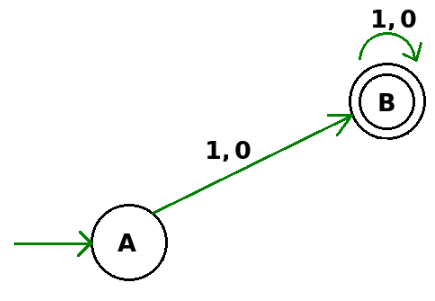So, the minimum number of states is 2.   Thus, B is the correct answer.

QUESTION: 4

Consider the following two statements:

I. If all states of an NFA are accepting states then the language accepted by the NFA is Σ∗ .
II. There exists a regular language A such that for all languages B, A ∩ B is regular.

Q. Which one of the following is CORRECT?

Solution:

Statement I : False, Since there is no mention of transition between states. There may be a case, where between two states there is no transition defined.
Statement II: True, Since any empty language (i.e., A = Φ ) is regular and its intersection with any other language is Φ. Thus A ∩ B is regular.

QUESTION: 5

In the automaton below, s is the start state and t is the only final state.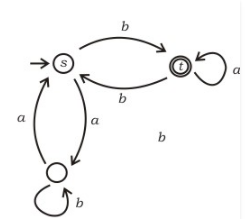Consider the strings u = abbaba, v = bab, and w = aabb. Which of the following statements is true?

Solution:

For the acceptance and rejection of any string we can simply check for the movement on each input alphabet between states. A string is accepted if we stop at any final state of the DFA. For string u=abbaba the string ends at t (final state) hence it is accepted by the DFA. For string v=bab the string ends at s (non-final state) and hence rejected by the DFA. For string w=aabb the string ends at s (non-final state) and hence rejected by the DFA.

QUESTION: 6

In the context-free grammar below, S is the start symbol, a and b are terminals, and ϵ denotes the empty string S → aSa | bSb | a | b | ϵ Which of the following strings is NOT generated by the grammar?

Solution:

The given Language is  Palindrome. A,C and D follow the language but  baba  is not a palindrome  , so B is the answer

QUESTION: 7

Which regular expression best describes the language accepted by the non-deterministic automaton below?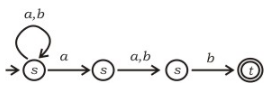Solution:
QUESTION: 8

Consider an ambiguous grammar G and its disambiguated version D. Let the language recognized by the two grammars be denoted by L(G) and L(D) respectively. Which one of the following is true ?

Solution:

By changing grammar, language will not change here. {as converting NFA to DFA language will not be changed}

QUESTION: 9

Which of the following regular expressions describes the language over {0, 1} consisting of strings that contain exactly two 1's?

Solution:

By looking at option A and D clearly not feasible solution.
Between B and C both contains exactly two 1's but in option B, both 1 will always come together where as in C it is general string.

QUESTION: 10

Let N be an NFA with n states and let M be the minimized DFA with m states recognizing the same language. Which of the following in NECESSARILY true?

Solution:

A state in a DFA is proper subset of states of NFA of corresponding DFA
=> set of states of NFA =n
=> no of subsets of a set with n elements = 2n
=> m<=2n

QUESTION: 11

If the final states and non-final states in the DFA below are interchanged, then which of the following languages over the alphabet {a,b} will be accepted by the new DFA?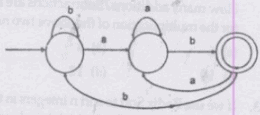Solution:

Seemingly, DFA obtained by interchanging final and non-final states will be complement of the given regular language. So, to prove that a family of string is accepted by complement of L, we will in turn prove that it is rejected by L.
(A). Set of all strings that do not end with ab - This statement could be proved right by looking at the b labeled incident edges on the final states and a labeled edges preceding them. Complement of the given DFA will have first two states as final states. First state doesn’t have any b labeled edge that has a labeled edge prior to it. Similarly, second final state doesn’t have any such required b labeled edge. Similarly, it could be proven that all the strings accepted by the given DFA end with ab. Now that L ∪ complement(L) = (a + b) ∗ , L should be the set of all the strings ending on ab and complement(L) should be set of all the strings that do not end with ab. [CORRECT]
(B). Set of all strings that begin with either an a or ab - This statement is incorrect. To prove that we just have to show that a string beginning with a or ab exists, which is accepted by the given DFA. String abaab is accepted by the given DFA, hence it won’t be accepted by its complement. [INCORRECT]
(C). Set of all strings that do not contain the substring ab - To prove this statement wrong, we need to show that a string exists which doesn’t contain the substring ab and is not accepted by current DFA. Hence, it will be accepted by its complement, making this statement wrong. String aba is not accepted by this DFA. [INCORRECT]
(D). The set described by the regular expression b ∗ aa ∗ (ba) ∗ b ∗ - String abaaaba is not accepted by the given DFA, hence accepted by its com

QUESTION: 12

Consider the following languages.

L1 = {ai bj ck | i = j, k ≥ 1}
L1 = {ai bj | j = 2i, i ≥ 0}

Q. Which of the following is true?

Solution:

A: Both L1 and L2 are CFL
B: L1 ∩ L2 = ∅ is true
L1is not regular => true
=> B is true
C: L1 ∪ L2 is not a CFL nut L2 is CFL is closed under Union
=> False
D: Both L1 and L2 accepted by DPDA

QUESTION: 13

Which of the following languages is (are) non-regular? L1 = {0m1n | 0 ≤ m ≤ n ≤ 10000} L2 = {w | w reads the same forward and backward} L3 = {w ∊ {0, 1} * | w contains an even number of 0's and an even number of 1's}

Solution:

1. L 1 is a regular language, as it can be derived by a trivial DFA with 10000 states for each alphabet in the grammar to limit on the number of 0s and 1s to 10000.
2. L 2 is a set of all palindromic strings, which isn’t a regular language because there is no way for a finite automaton to remember which alphabets have occurred.
3. L 3 is a standard regular language as there exists a DFA which can derive this language. You can read more in the references about it.

QUESTION: 14

Consider the following two finite automata. M1 accepts L1 and M2 accepts L2.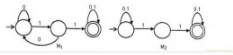Q. Which one of the following is TRUE?

Solution: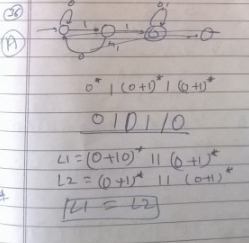QUESTION: 15

Which of the following intuitive definition is true about LR(1) Grammar.

Solution:

LR(1) – Reading the input string from left to right.
LR(1) – Deriving a right-most derivation for the string.
In the first choice we read from Left to right deriving the right-most sentential form and looking one symbol ahead which is stack top. So option (a) is correct.

QUESTION: 16

Consider the Following regular expressions

r1 = 1(0 + 1)*
r2 = 1(1 + 0)+
r3 = 11*0

Q. What is the relation between the languages generated by the regular expressions above ?

Solution:

Clearly r1 is a superset of both r2 and r3 as string 1 can not be generated by r2 and r3. r2 is a superset of r3 as string 11 is not present in L(r3) but in L(r2).

QUESTION: 17

Consider regular expression r, where r = (11 + 111)* over Ʃ = {0, 1}. Number of states in minimal NFA and DFA respectively are:

Solution: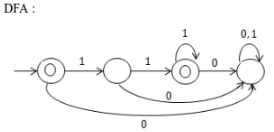For NFA: Just remove the trap state.

QUESTION: 18

Consider the grammar G.

E -> TE’
E’ -> +TE’ | ԑ
T’ -> FT’
T’ -> *FT’ | ԑ
F -> (E) | id

If LL(1) parsing table is constructed using the grammar G, then how many entries are present in the row that represents E’ nonterminal ? (consider the entries which are not error/not blank entries)

Solution:

First (E’) = {+ ,ԑ} Follow (E’) = {\$ , )}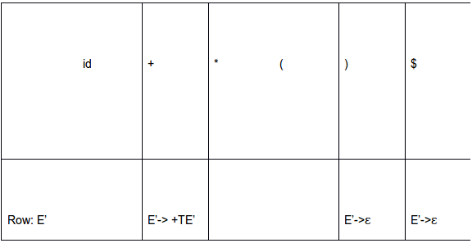Therefore 3 entries are present in E’ row

QUESTION: 19

Let, init (L) = {set of all prefixes of L},
Let L = {w | w has equal number of 0’s and 1’s}
init (L) will contain:

Solution:

Clearly init (L) = (0+1)*. Take any string in (0+1)* say 101011, we can append required number of symbols to make it a member of L like 10101100.

QUESTION: 20

Suppose M1 and M2 are two TM’s such that L(M1) = L(M2). Then

Solution:

M2 accepts strings in L(M1) so definitely it will halt. Other 2 are not guaranteed as Turing machine M does not accept w if it either rejects w by halting to a reject state or loops infinitely on w.